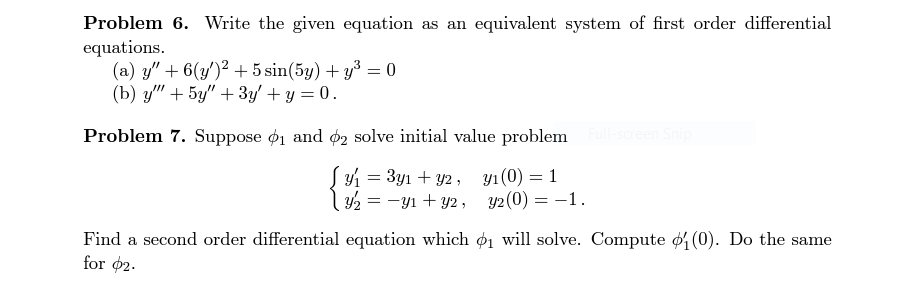# Question Problem 6. Write the given equation as an equivalent system of first order differential equations. (a) $$y^{\prime \prime}+6\left(y^{\prime}\right)^{2}+5 \sin (5 y)+y^{3}=0$$ (b) $$y^{\prime \prime \prime}+5 y^{\prime \prime}+3 y^{\prime}+y=0$$. Problem 7. Suppose $$\phi_{1}$$ and $$\phi_{2}$$ solve initial value problem $\left\{\begin{array}{l} Problem 6. Write the given equation as an equivalent system of first order differential equations. (a) $$y^{\prime \prime}+6\left(y^{\prime}\right)^{2}+5 \sin (5 y)+y^{3}=0$$ (b) $$y^{\prime \prime \prime}+5 y^{\prime \prime}+3 y^{\prime}+y=0$$. Problem 7. Suppose $$\phi_{1}$$ and $$\phi_{2}$$ solve initial value problem \[ \left\{\begin{array}{l} y_{1}^{\prime}=3 y_{1}+y_{2}, \quad y_{1}(0)=1 \\ y_{2}^{\prime}=-y_{1}+y_{2}, \quad y_{2}(0)=-1 \end{array}\right.$ Find a second order differential equation which $$\phi_{1}$$ will solve. Compute $$\phi_{1}^{\prime}(0)$$. Do the same for $$\phi_{2}$$.Transcribed Image Text: Problem 6. Write the given equation as an equivalent system of first order differential equations. (a) $$y^{\prime \prime}+6\left(y^{\prime}\right)^{2}+5 \sin (5 y)+y^{3}=0$$ (b) $$y^{\prime \prime \prime}+5 y^{\prime \prime}+3 y^{\prime}+y=0$$. Problem 7. Suppose $$\phi_{1}$$ and $$\phi_{2}$$ solve initial value problem $\left\{\begin{array}{l} y_{1}^{\prime}=3 y_{1}+y_{2}, \quad y_{1}(0)=1 \\ y_{2}^{\prime}=-y_{1}+y_{2}, \quad y_{2}(0)=-1 \end{array}\right.$ Find a second order differential equation which $$\phi_{1}$$ will solve. Compute $$\phi_{1}^{\prime}(0)$$. Do the same for $$\phi_{2}$$.
Transcribed Image Text: Problem 6. Write the given equation as an equivalent system of first order differential equations. (a) $$y^{\prime \prime}+6\left(y^{\prime}\right)^{2}+5 \sin (5 y)+y^{3}=0$$ (b) $$y^{\prime \prime \prime}+5 y^{\prime \prime}+3 y^{\prime}+y=0$$. Problem 7. Suppose $$\phi_{1}$$ and $$\phi_{2}$$ solve initial value problem $\left\{\begin{array}{l} y_{1}^{\prime}=3 y_{1}+y_{2}, \quad y_{1}(0)=1 \\ y_{2}^{\prime}=-y_{1}+y_{2}, \quad y_{2}(0)=-1 \end{array}\right.$ Find a second order differential equation which $$\phi_{1}$$ will solve. Compute $$\phi_{1}^{\prime}(0)$$. Do the same for $$\phi_{2}$$.